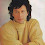Mathematics teaching learning लाई popular बनाउन

1. घोकाएर,रटाएर पढाउने कुरालाई निरुत्साहित गर्नुपर्छ
2. conceptदिएर सिकाउनु पर्छ
3. Mathematics meaningful बनाउनुपर्छ ।
4. Mathematics लाई जीवनसित जोडेर teaching learning क्रियाकलाप गर्नुपर्छ।

यसको लागी

1. Digital MATHLAB को प्रयोग सुरु गर्नुपर्छ।
2. Digital toolको प्रयोग सुरु गर्न  teacher लाई  empower गर्नुपर्छ।
3. Student लाई  सामग्री चलाउन लगाएर,  concept स्पष्ट पारेर, mathematics लाई जीवनसित जोडेर teaching learning क्रियाकलाप गर्नुपर्छ।
4. Mathematical results को  meaning र interpretation को बारेमा छलफल गर्नुपर्छ
Digital MATHLAB को एउटा सामग्री र यसको प्रयोग तल उल्लेख गरिएको छ।

1. Mouse ले Set Region मा Click गर्नुहोस
2. नयाँ प्रश्नको लागी New Question मा  Click गर्नुहोस

## HOW TO USE IT IN THE CLASSROOM

Introduction
This lesson is designed to introduce students to the idea of a set with Venn-Diagram. In this Lesson, Students will experiment with sets in conjunction with the Venn Diagram.

Objectives
Upon completion of this lesson, students will:
understand the notion of sets in Venn diagrams.
learn the terminology used with sets and Venn diagrams.
practice the placement of set notion in a Venn Diagram.

Activities
Open the link in order to demonstrate this activity to the students.

Begin to explain the applet to the students by showing them the first example on the page. Ask the class if they know what the answer is.
When a student has responded correctly, show the class that by clicking in the appropriate section of the diagram, the circles representing the sets.

Show the students the location of the "Check Answer" button and check the students' answer together.

Guided Practice
Let students to try another example, letting the students direct your moves. Or, you may simply ask, "Can anyone describe the answer?"

Independent Practice
Allow the students to work on their own and to complete some homework.

HOW TO PREPARE
Design the sets

A: x² + y² ≤ 9
B: (x - 3)² + y² ≤ 9
U: -5 ≤ x ≤ 8 ∧ -4 ≤ y ≤ 4
AUB: x² + y² ≤ 9 ∨ (x - 3)² + y² ≤ 9
Q:=Polygon((-5, 4), (8, 4), (8, -4), (-5, -4), (-5, 4))
Hide A,B,U, AUB

Design the Regions
c1: x² + y² = 9
c2: (x - 3)² + y² = 9
part1: x² + y² ≤ 9 ∧ (¬((x - 3)² + y² ≤ 9))
part2: (¬(x² + y² ≤ 9)) ∧ (x - 3)² + y² ≤ 9
part3: x² + y² ≤ 9 ∧ (x - 3)² + y² ≤ 9
part4: -5 ≤ x ≤ 8 ∧ -4 ≤ y ≤ 4 ∧ (¬(x² + y² ≤ 9 ∨ (x - 3)² + y² ≤ 9))

Design the logics
IsRed1 = true
IsRed2 = true
IsRed3 = true
IsRed4 = true
RB1:=If(IsRed1, 0, 1)
RB2:=If(IsRed2, 0, 1)
RB3:=If(IsRed3, 0, 1)
RB4:=If(IsRed4, 0, 1)
R1:=If(IsRed1, 0.9, 1)
R2:=If(IsRed2, 0.9, 1)
R3:=If(IsRed3, 0.9, 1)
R4:=If(IsRed4, 0.9, 1)

Setup the Objective Properties
part 1

Dynamic Colors
Red R1
Green RB1
Blue RB1
Opacity 0.75
Scripting
On Click
SetValue[IsRed1,¬IsRed1]

part 2

Dynamic Colors
Red R2
Green RB2
Blue RB2
Opacity 0.75
Scripting
On Click
SetValue[IsRed2,¬IsRed2]

part 3

Dynamic Colors
Red R3
Green RB3
Blue RB3
Opacity 0.75
Scripting
On Click
SetValue[IsRed3,¬IsRed3]

part 4

Dynamic Colors
Red R4
Green RB4
Blue RB4
Opacity 0.75
Scripting
On Click
SetValue[IsRed4,¬IsRed4]

Define the logic for score

 A_0 1 B_0 2 AΔB 3 AB 4 A 5 B 6 AUB 7 (AUB)' 8 B' 9 A' 10 (A∩B)' 11 (AΔB)' 12 B_0' 13 A_0' 14 U 15 Φ 0

score:=IsRed1 + 2IsRed2 + 4IsRed3 + 8IsRed4

Define the problems
problem = {{"A_0",1},{"B_0",2},{"AΔB",3},{"A∩B",4},{"A",5},{"B",6},{"AUB",7},{"(AUB)'",8},{"B'",9},{"A'",10},{"(A∩B)'",11},{"(AΔB)'",12},{"B_0'",13},{"A_0'",14},{"U",15},{"Φ",0}}

Define item
max:=Length(problem)
item:=Slider(1, max, 1)

Define the key
key:=Element(problem, item, 2)

Define a Button for New Question
Text New Question
Scripting
On Click
SetValue[item,RandomBetween[1,max]]

Define two texts for output

Congratulation
Condition to Show Object
score ≟ key

Try Again
Condition to Show Object
score ≠ key

Define the name plate
Text A
Text B
Text U
Text Shade the red color for the set

Define Question text
name Question
Definition Element(problem, item, 1)

1.It is Such a good article
Keep Motivating us on giving these nice articles... Wolfram Mathematica

2.Your Blog is quite engrossing and I hope your blog is beneficial for viewers.
https://hitplugins.com/turbotax-activation-code-crack/

3.